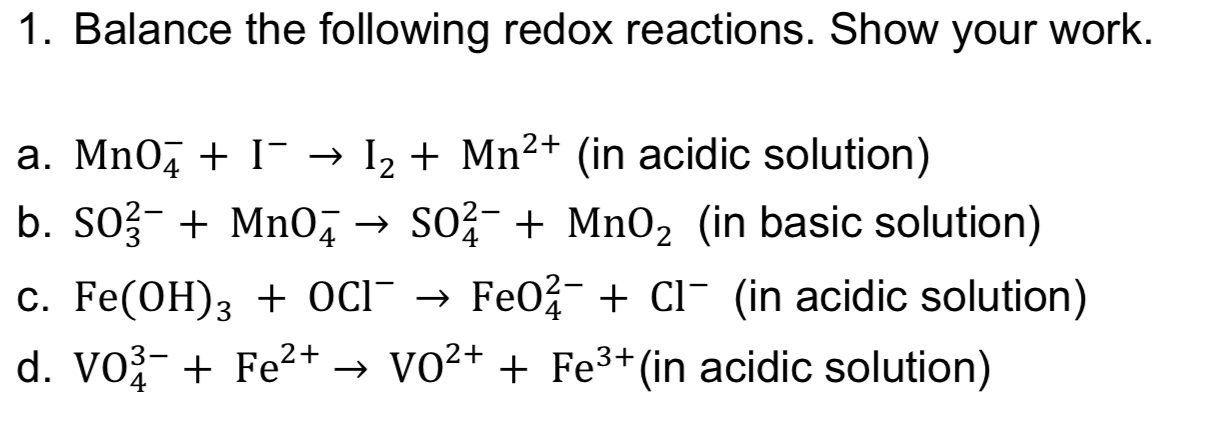# 1. Balance the following redox reactions. Show your work.Mn2+ (in acidic solution)Ia. Mn0I2b. SO3 Mn04SO2Mn02 (in basic solution)Fe02 CI (in acidic solution)C. Fe(OH)3 + OcI -d. VO3 Fe2+ -> VO2+ + Fe3+ (in acidic solution)

Question
10 viewshelp_outlineImage Transcriptionclose1. Balance the following redox reactions. Show your work. Mn2+ (in acidic solution) I a. Mn0 I2 b. SO3 Mn04SO2Mn02 (in basic solution) Fe02 CI (in acidic solution) C. Fe(OH)3 + OcI - d. VO3 Fe2+ -> VO2+ + Fe3+ (in acidic solution) fullscreen
check_circle

Step 1

Since, the question you have posted requires lengthy steps for the solution, so we are providing the answer of the first question, that is 1. (a) only. If you require the answers for the other parts, please post the questions again separately.

Step 2

1.(a) MnO4- + I- → Mn2+ + I2 (in acidic solution)

The balancing of this reaction equation in the acidic medium can be done by the half reaction method. The various steps involved in the balancing of this chemical equation are as follows:

Step1: write the oxidation and reduction half reactions and indicate the oxidation number on each atom.

Step 3

Step 2: balance the number of atoms if req...

### Want to see the full answer?

See Solution

#### Want to see this answer and more?

Solutions are written by subject experts who are available 24/7. Questions are typically answered within 1 hour.*

See Solution
*Response times may vary by subject and question.
Tagged in

### Redox Reactions